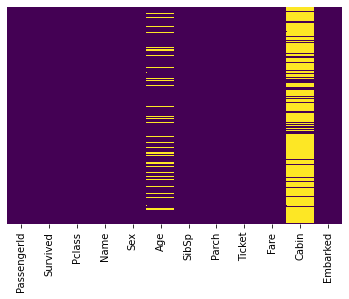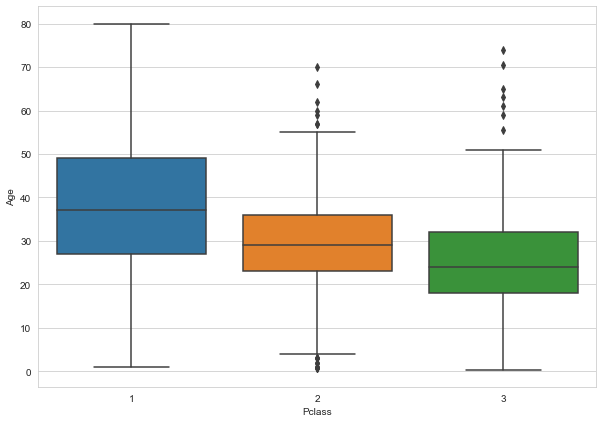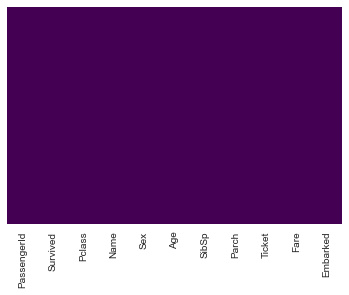A great simple example on how to deal with Logistic Regression in Python utilizing Matplotlib, Seaborn, and Scikit-learn. The data is pulled from Kaggle.com and found here. Normally this comes as a test and train set, but I’m analyzing the training set only to see the accuracy of the model.

The data here is a little dirty and necessitated some data cleansing first, probably worthy of another short tutorial / post in terms of some other methods of initial cleansing before working on data…

# Logistic Regression - Simple Example¶

## I.E. Binary choice comparison¶

Utilizes the Titanic Dataset from Kaggle.com. We have a training set and a testing set (CSV), but I'm only working on the training set as if it's the full set - showing how to split that into testing/training at the end. This will allow us to see the accuracy of the model

We'll walk through exploring, cleansing, and applying regression to this dataset

In :
```#Initial Imports
import pandas as pd
import numpy as np
import matplotlib.pyplot as plt
import seaborn as sns
```
In :
```#Import training data
```

#### Exploratory¶

In :
```titanic.head()
```
Out:
PassengerId Survived Pclass Name Sex Age SibSp Parch Ticket Fare Cabin Embarked
0 1 0 3 Braund, Mr. Owen Harris male 22.0 1 0 A/5 21171 7.2500 NaN S
1 2 1 1 Cumings, Mrs. John Bradley (Florence Briggs Th... female 38.0 1 0 PC 17599 71.2833 C85 C
2 3 1 3 Heikkinen, Miss. Laina female 26.0 0 0 STON/O2. 3101282 7.9250 NaN S
3 4 1 1 Futrelle, Mrs. Jacques Heath (Lily May Peel) female 35.0 1 0 113803 53.1000 C123 S
4 5 0 3 Allen, Mr. William Henry male 35.0 0 0 373450 8.0500 NaN S
In :
```#We have some missing data here, so we're going to map out where NaN exists
#We might be able to take care of age, but cabin is probably too bad to save
sns.heatmap(titanic.isnull(),yticklabels=False,cbar=False,cmap='viridis')
```
Out:
`<matplotlib.axes._subplots.AxesSubplot at 0x1b524f50d48>`#### Clean the missing data¶

You could jump to the end if you already have clean data, this one is just a bit messy

In :
```#Determine the average ages of passengers by class
#In an attempt to fix the NaN for this column somewhat
sns.set_style('whitegrid')
plt.figure(figsize=(10,7))
sns.boxplot(x='Pclass',y='Age',data=titanic)
```
Out:
`<matplotlib.axes._subplots.AxesSubplot at 0x1b523ddb208>`In :
```#Fill out the Age column with the average ages of passengers per class
def impute_age(cols):
Age = cols
Pclass = cols

if pd.isnull(Age):
if Pclass == 1:
return 37 #Return the avg age of passengers in the 1st class
elif Pclass == 2:
return 29 #2nd class
else:
return 24 #3rd class
else:
return Age

#Apply the function to the Age column
titanic['Age'] = titanic[['Age','Pclass']].apply(impute_age,axis=1)

#Drop the cabin data (plus anything else) since it's unsalvagable / not a big deal
titanic.drop('Cabin',axis=1,inplace=True)
titanic.dropna(inplace=True)
```
In :
```#Recheck the heatmap
#No more problems with Age
sns.heatmap(titanic.isnull(),yticklabels=False,cbar=False,cmap='viridis')
```
Out:
`<matplotlib.axes._subplots.AxesSubplot at 0x1b52596d1c8>`#### Other data cleansing, Parse down dataset¶

In :
```#Since Sex and Embark are columns that are perfect predictors of one another (Male of 0 always means 1 of Female)
#We merge these into single columns
sex = pd.get_dummies(titanic['Sex'],drop_first=True)
embark = pd.get_dummies(titanic['Embarked'],drop_first=True)
```
In :
```#Add the new columns to the dataset
titanic = pd.concat([titanic,sex,embark],axis=1)
```
In :
```#Drop the columns we can't use
titanic.drop(['PassengerId','Sex','Embarked','Name','Ticket'],axis=1,inplace=True)
```
In :
```titanic.head()
```
Out:
Survived Pclass Age SibSp Parch Fare male Q S
0 0 3 22.0 1 0 7.2500 1 0 1
1 1 1 38.0 1 0 71.2833 0 0 0
2 1 3 26.0 0 0 7.9250 0 0 1
3 1 1 35.0 1 0 53.1000 0 0 1
4 0 3 35.0 0 0 8.0500 1 0 1

#### Run Machine Learning Algorithm¶

Utilizing the training set ONLY and split it (pretending we don't have two CSV files already)

Determine survival

In :
```#Assign variables
X = titanic.drop('Survived', axis=1)
y = titanic['Survived']
```
In :
```from sklearn.model_selection import train_test_split
```
In :
```#Choose the test size
#Test size = % of dataset allocated for testing (.3 = 30%)
#Random state = # of random splits
X_train, X_test, y_train, y_test = train_test_split(X, y, test_size=0.3, random_state=101)
```
In :
```from sklearn.linear_model import LogisticRegression
```
In :
```#Object, added max_inter since I hit a limit later
logmodel = LogisticRegression(max_iter=10000)
```
In :
```#Fit model
logmodel.fit(X_train,y_train)
```
Out:
`LogisticRegression(max_iter=10000)`
In :
```#Form predictions
predictions = logmodel.predict(X_test)
```
In :
```from sklearn.metrics import classification_report
from sklearn.metrics import confusion_matrix
```
In :
```#Simple report
print(classification_report(y_test,predictions))
```
```              precision    recall  f1-score   support

0       0.82      0.92      0.87       163
1       0.85      0.69      0.76       104

accuracy                           0.83       267
macro avg       0.84      0.81      0.82       267
weighted avg       0.83      0.83      0.83       267

```
In :
```#Or print out a confusion matrix
print(confusion_matrix(y_test,predictions))
```
```[[150  13]
[ 32  72]]
```